# Category Archives: CoCo

Tandy/Radio Shack TRS-80 Color Computer (CoCo)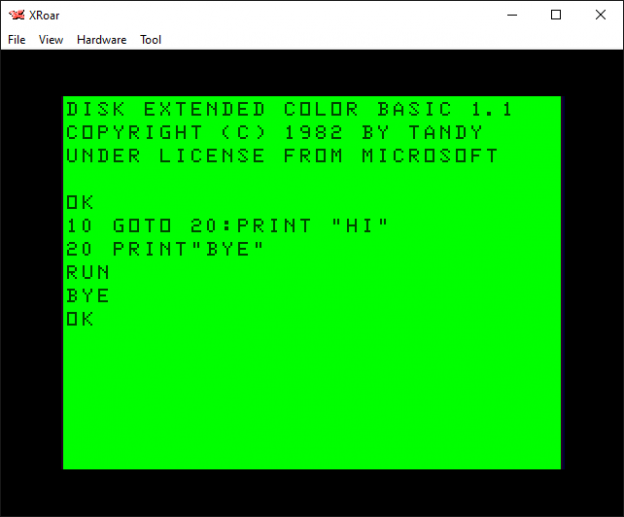# What ELSE can you do when you don’t have ELSE?

NOTE: I have a follow-up to this ELSE thing already written which “changes everything” for me. There’s stuff about ELSE I never knew, which makes me want to never use it. More on that in the next installment. And now back to your regularly scheduled blog post…

First, a quick BASIC memory saving tidbit.

Shaun Bebbington left a comment about my VIC-20 dissection article asking if I knew you could omit zeros from DATA statements like this:

`100 DATA 128,0,0,50,239,0,123,42,0,4`

If you leave them out (comma comma), READ will still get a zero! It saves a byte each time you do that, which could be important on a machine with 3584 bytes free on startup like my VIC-20.

`100 DATA 128,,,50,239,,123,42,,4`

Good tip, Shaun! Thanks!

And while I had his attention, I asked him a few Commodore BASIC questions. I was wondering how you did IF/THEN/ELSE without ELSE! One of the many things I liked about the BASIC in the CoCo was it had ELSE and commands like PLAY, DRAW, CIRICLE, etc. My VIC-20 had no ELSE. In my Sky-Ape-Er game, I’d see my young self doing things like this:

```125 IF K=17 THEN L=L-1:M=14
130 IF K=41 THEN L=L+1:M=13
135 IF K=39 THEN 180```

In that code snippet, K represented the key value that was pressed. If it was 17 (left), I’d move the player left one and change its graphic character. If it was 41 (right), I’d move the player right one and change its graphics character. If it was 39 (F1, jump), I’d go to a routine that would handle the jump.

Without ELSE, if K was 17, it would do that, then check K two more times, even though it didn’t need to. Maybe I did not “see” it back then, but If I had the bytes to spare, I probably should have done something like this:

```125 IF K=17 THEN L=L-1:M=14:GOTO 140
130 IF K=41 THEN L=L+1:M=13:GOTO 140
135 IF K=39 THEN 180```

That way, if K=17 was true, it would do it’s thing, then GOTO would skip over the next two lines. This could be a huge time saver if there were a bunch of conditions (up, down, left, right, diagonals, jump, fire, punch, windshield wipers, etc.)

Someone has already suggested that I may have done it my original way to get consistent timing in the game loop. Somehow I doubt my junior high self was that clever. But I digress…

With ELSE, it could have been written like this:

`125 IF K=17 THEN L=L-1:M=14 ELSE IF K=41 THEN L=L+1:M=13 ELSE IF K=39 THEN 180`

Less code (the ELSE token takes up less memory than an addition line number and/or the extra GOTO to skip lines), and faster execution, maybe.

Side Note: Maybe? There is still the issue of, when completing a successful IF, BASIC’s parser still having to run through the rest of the line characters to find the end and the next line. Adding a “GOTO” wouldn’t help, either, since it would have to parse that and then STILL have to scan to the end of the line. (At least on Color BASIC, which does not remember line pointers once it is parsing a line.) It may actually be faster to break up a long set of IF/ELSE into small lines with a GOTO.

But Shuan mentioned a way of using ON to simulate an ELSE. A quick search led me to a forum post discussing this very thing.

It supposedly works like this… since a compare (K=42, A\$=”RED”, G>100) will return either -1 (false) or 0 (true), you can do an ON GOTO based on the result of that compare. Since it’s a -1 or 0, you can just make the result negative (thus, -1 becomes 1, and 0 becomes -0 which is still 0):

```10 ON -(A=42) GOTO 20:PRINT "NOT 42":END
20 PRINT "42!"```

Er… what? I thought BASIC did not execute anything after a GOTO. It doesn’t, does it?

But… but… How can ON/GOTO with something after it work, then? It turns out, ON/GOTO is special since the conditions of the “ON” may not be met, and thus the GOTO part may not get executed and BASIC will continue.

`ON A GOTO 100,200,300:PRINT "A WAS NOT 1, 2 or 3"`

Looking at it like that makes perfect sense to me. If A is not 1, 2 or 3, it won’t GOTO anywhere and the line continues to be processed.

Thus, this odd code serves as a simple “ELSE” when you don’t have ELSE:

```10 INPUT "VALUE";A
20 ON -(A=42) GOTO 30:GOTO 10
30 PRINT "YOU KNOW THE ANSWER!"```

…would be like…

```10 INPUT "VALUE";A
20 IF A=42 THEN 30 ELSE 10
30 PRINT "YOU KNOW THE ANSWER!"```

Interesting! I have not benchmarked this to see if it’s faster than using GOTO to skip lines, but it might be smaller due to not needing another few bytes for each line number.

Would this have helped my “left, right, jump” code? Maybe not. You can string these together like this:

```124 ON -(K=17) GOTO 125:ON -(K=42) GOTO 130:ON -(K=39) GOTO 180
125 L=L-1:M=14:GOTO 140
130 L=L+1:M=13:GOTO 140```

Since I was doing code in response to the IF, the ON/GOTO approach would just let me GOTO a line that does that code, which then still needs a GOTO to skip the lines after it that shouldn’t be executed. Not great for that use.

But, if I were dispatching to routines based on direction (like I do with the “jump” check to line 180), it would have worked just fine. Instead of this:

```125 IF K=17 THEN 200
130 IF K=41 THEN 300
135 IF K=39 THEN 400
...
200 REM Handle LEFT
...
300 REM Handle RIGHT
...
400 REM Handle JUMP
...```

Those three lines could be combined into one like this:

`124 ON -(K=17) GOTO 200:ON -(K=42) GOTO 300:ON -(K=39) GOTO 400`

But, doing a quick test typing in JUST those lines on a VIC-20 emulator, I got 3530 bytes free after each approach. No penalty, but no savings, and all the parsing with parens and the negatives is probably slower.

Interesting, for sure. Useful? I guess I’ll find out if I get around to updating my VIC-20 code.

## Bonus offer

I also saw this example in the Commodore forum:

```10 rem if then else demo
20 input a
30 if a = 1 then goto 60
40 print "this is the else part"
50 goto 70
60 print "this is the if (true) part"
70 end```

This is how I’d write that with ELSE:

```20 INPUT A
30 IF A=1 THEN PRINT "THIS IS THE IF (TRUE) PART" ELSE PRINT "THIS IS THE ELSE PART"```

So we could change the Commodore example to match this a bit closer:

```20 input a
30 if a = 1 then print "this is the if (true) part":goto 70
40 print "this is the else part"
70 end```

…which leads us back to just adding a GOTO at the end of each separate IF:

```10 GET A\$:IF A\$="" THEN 10
20 IF K\$="U" THEN Y=Y-1:GOTO 70
30 IF K\$="D" THEN Y=Y+1:GOTO 70
40 IF K\$="L" THEN X=X-1:GOTO 70
50 IF K\$="R" THEN X=X+1:GOTO 70
60 GOTO 10
70 REM DRAW X,Y...
80 GOTO 10```

…which on the CoCo’s Extended Color BASIC might look like:

```10 GET A\$:IF A\$="" THEN 10
20 IF K\$="U" THEN Y=Y-1 ELSE IF K\$="D" THEN Y=Y+1 ELSE IF K\$="L" THEN X=X-1 ELSE IF K\$="R" THEN X=X+1 ELSE 10
70 REM DRAW X,Y...
80 GOTO 10```

Ultimately, we are just trading “ELSE” with “GOTO xx” and a new line, with ELSE being smaller due it it just being a token, verses the overhead of a GOTO token and the line number characters after it, AND a new line for each additional “else” condition.

Until next time, ELSE maybe sooner…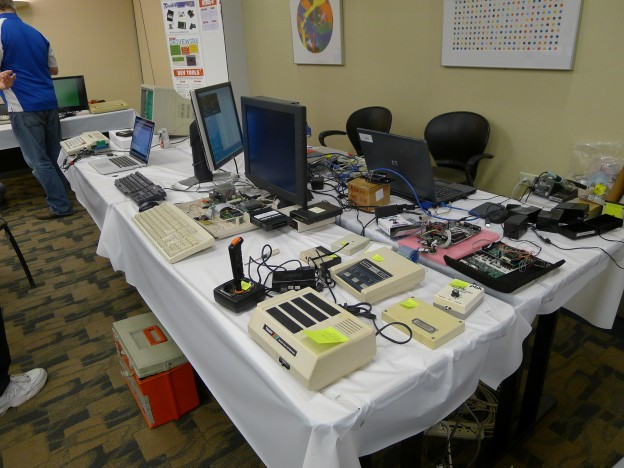# Virtual CoCoFest this Saturday, April 18, 2020.

News release:

## CoCoTALK! is hosting a live, virtual CoCoFEST!

April 18th, 2020 would have been the 29th annual “last” Chicago CoCoFEST! celebrating the 40th anniversary of the Tandy RadioShack TRS-80 Color Computer. While the event itself has been postponed, the live retro podcast CoCoTALK! is hosting a live, virtual CoCoFEST! Saturday April 18th @ 2:00 PM EDT.You can view and chat live on YouTube, the friendly link is http://live.cocotalk.live which points to  https://www.youtube.com/c/Ogsteviestrow/live

CoCoFEST! is an annual event hosted by the Glenside Color Computer Club and has been running since the last Rainbow Fest ended.  This event is the K-FEST/VCF event of the Color Computer world. The virtual event is not an official Glenside Color Computer event, but is  being held, with their approval.  The goal is to get as many of the attendees, exhibitors, vendors, speakers, and presenters to join the live call present virtually, and turn the event into one that can be experience globally.

CoCoTALK! is a weekly live and interactive video talk show which multicast to YouTube and Facebook live, so there will be plenty of ways to join the celebration with us.  To learn more about CoCoTALK! go to http://cocotalk.live To learn more about the Glenside Color Computer club, visit http:///glensideccc.com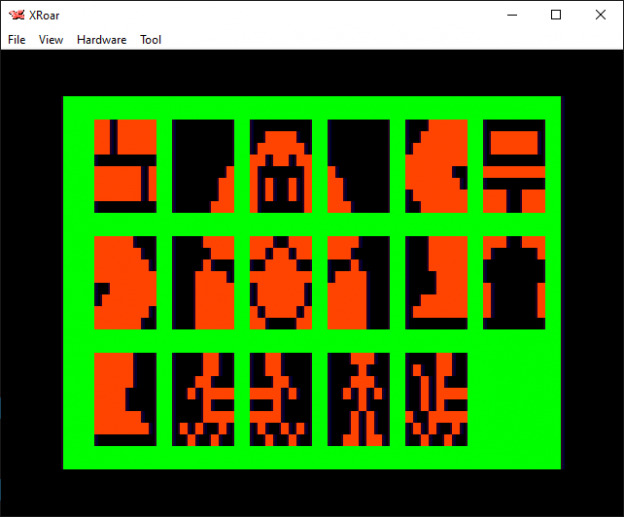# VIC-20: Sky-Ape-Er code dissection – part 2

See also: part 1, part 2, part 3, part 4 or part 5 (with more coming).

Welcome to my second VIC-20 Tuesday!

Previously, I began to dissect my old VIC-20 game Sky-Ape-Er. It was made up of two BASIC programs:

1. INSTRUCTIONS – Display instructions, set up custom character set, then load and run the main game.
2. SKY-APE-ER – The main game.

## VIC-20 custom fonts

The VIC-20 did not have a true graphics screen. Instead, it used font characters that could be dynamically changed. Each character on the 22×20 screen was 8×8. You could create custom 8×8 characters to represent anything you wanted.

I believe I used a program called Eight by Eight Create by Robert Spahitz from the January 1983 issue of Creative Computing (Vol 9, Number 1). See page 270 (or 272 of this PDF scan):

https://archive.org/details/creativecomputing-1983-01/page/n271/mode/2up

Or this text version of just the article.

This also helps me confirm that I got my VIC-20 in 1982 since I initially did not have a Commodore Datasette tape deck for it and had no way to save programs until later. By the time this issue came out, I had the tape deck.

But I digress…

## VIC-20 custom fonts on the CoCo

I have screen shots of what the custom characters looked like in the VIC-20 game, but thought it would be fun to take those DATA statements and display them on my CoCo. Using lines 100 to 125 from the INSTRUCTIONS program, I created this Color BASIC program:

```0 REM skychars.bas
5 POKE 65495,0
10 CLS:XO=4:YO=2
15 DIM BM(7):FOR A=0 TO 7:BM(7-A)=(2^A):NEXT
20 FOR R=0 TO 7
25   READ V:IF V=-1 THEN 99
30   FOR C=0 TO 7
35     IF V AND BM(C) THEN SET(XO+C,YO+R,8) ELSE RESET(XO+C,YO+R)
40   NEXT
45 NEXT
50 XO=XO+10:IF XO>60 THEN XO=4:YO=YO+10
55 GOTO 20
99 GOTO 99
100 DATA223,223,223,0,253,253,253,0,0,0,0,0,1,3,3,7,0,60,126,219,129,165,165,129
105 DATA0,0,0,0,128,192,192,224,31,63,127,255,252,254,127,63,0,126,126,0,255,0,231,231
110 DATA248,252,254,255,63,127,254,252,15,7,8,30,31,31,31,31,231,219,60,255,126,126
115 DATA189,195,240,224,16,120,248,248,248,248,31,31,31,15,15,63,127,0,231,129,0,0,129
120 DATA129,129,0,248,248,248,240,240,252,254,0,12,12,24,47,8,15,82,36,48,48,24,244,16
125 DATA240,74,36,28,8,28,42,8,20,20,54,12,76,40,47,40,159,82,36
130 DATA -1```

It now displays what those font characters look like:

These custom characters would replace “@ABCDEFGHIJKLMNOP”. The “@” symbol was the brick used to make the game playfield.

Letters A-L were used to make the ape, like this:

```ABC
DEF
GHJ
JLK```

Letters M-O were the player’s character, facing right, left and forward.

Letter P were the small apes that were running towards the player. They never turned back, so no additional characters we needed for them.

My program was written to just put those characters on the screen, which would look like this:

But with the custom font in use, it looks like this:

In the next installment, I’ll walk through the actual SKY-APE-ER game code and see how it works.

Until next time…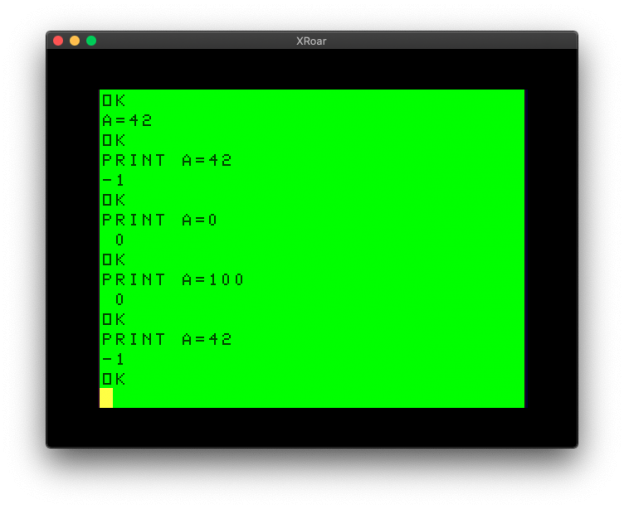# IF AND/OR THEN versus IF THEN IF

## Or, “I’ll NEVER compare multiple values the same way again”

Here is a quick Optimizing Color BASIC quickie.

Tonight, I saw this YouTube video from Robin at 8-Bit Show and Tell. He was responding to someone who noticed a bit of code in an old Commodore magazine that did:

`IF Z>192 THEN IF Z<219 THEN Z=Z-128`

`IF Z>192 AND Z<219 THEN Z=Z-128`

He performed a benchmark very much like the ones I have been doing on the CoCo (I’m glad I’m not the only one doing this). I decided to try it on the CoCo and see what happened here.

I first tried the normal “IF AND THEN” way:

```0 REM IFAND.BAS
5 DIM TE,TM,B,A,TT
10 FORA=0TO3:TIMER=0:TM=TIMER
20 FORB=0TO1000
30 IF Z>192 AND Z<219 THEN Z=Z-128
70 NEXT
80 TE=TIMER-TM:PRINT,TE
90 TT=TT+TE:NEXT:PRINTTT/A:END
```

This produced 875 on my Xroar emulator.

Then I tried the “odd” IF AND THEN way…

```0 REM THENIF.BAS
5 DIM TE,TM,B,A,TT
10 FORA=0TO3:TIMER=0:TM=TIMER
20 FORB=0TO1000
30 IF Z>192 THEN IF Z<219 THEN Z=Z-128
70 NEXT
80 TE=TIMER-TM:PRINT,TE
90 TT=TT+TE:NEXT:PRINTTT/A:END```

This produced 472! A significant time savings just by changing the way you compare multiple things.

Thinking this through, I have some theories, based on some very inefficient C code I found in a work project once.

See A C tale of two ampersands for that story…

Could BASIC be doing the same thing?

## IF THEN BASIC

“IF this AND that THEN” surely isn’t doing a logical AND for values, is it? It might be, since you can print a comparison like this:

In Color BASIC, true is -1 and false is 0. So perhaps doing this:

`IF A=1 AND B=2 THEN PRINT "BOTH ARE TRUE"`

…is actually turning into…

`IF -1 AND 0 THEN PRINT "BOTH ARE TRUE"`

…with -1 or 0 being used based on the conditions. Let’s try:

Oh my. Maybe it IS doing this, thus requiring the values to be saved and compared, making it slower just like C generating more code to do the same thing.

I know I could just look at the disassembly in Color BASIC Unravelled and know exactly what is going on, but it’s more fun to experiment. How can we test this?

Well, things that return true/false return -1 or 0. You can AND two numbers together and get mathematical results. Even a compare like:

`IF A\$="ALLEN" THEN`

…will return a -1 if it is true, or a 0 if it is false:

It sure seems likely that BASIC is just taking the result of a compare (true or false, -1 or 0) and AND-ing or OR-ing those values. Thus, my theory is this:

Multiple AND/OR comparisons turn into multiple results of -1 or 0 (true or false) which are then mathematically processed:

`IF A=1 AND B=2 AND C=3 THEN ...`

…so all of it has to be processed. But, using this IF THEN IF approach:

`IF A=1 THEN IF B=2 THEN IF C=3 THEN ...`

…will be much faster since it does a compare, then if good, it does another compare, then if good, it does the final compare… rather than having to do three compares then do math on the three results of that compare then check that final result to know what to do.

What says you?

I suppose it gets much slower the more conditions you want to compare:

```5 DIM TE,TM,B,A,TT
10 FORA=0TO3:TIMER=0:TM=TIMER
20 FORB=0TO1000
30 IF X=1 AND Y=1 AND Z=1 THEN Z=42
70 NEXT
80 TE=TIMER-TM:PRINT,TE
90 TT=TT+TE:NEXT:PRINTTT/A:END```

763 for that one. Versus:

```5 DIM TE,TM,B,A,TT
10 FORA=0TO3:TIMER=0:TM=TIMER
20 FORB=0TO1000
30 IF X=1 THEN IF Y=1 THEN IF Z=1 THEN Z=42
70 NEXT
80 TE=TIMER-TM:PRINT,TE
90 TT=TT+TE:NEXT:PRINTTT/A:END```

335!

So obviously I have to do this:

```5 DIM TE,TM,B,A,TT
10 FORA=0TO3:TIMER=0:TM=TIMER
20 FORB=0TO1000
30 IF G=1 AND H=1 AND I=1 AND J=1 AND K=1 AND L=1 AND M=1 AND N=1 AND O=1 AND P=1 AND Q=1 AND R=1 AND S=1 AND T=1 AND U=1 AND V=1 AND W=1 AND X=1 AND Y=1 AND Z=1 THEN Z=42
70 NEXT
80 TE=TIMER-TM:PRINT,TE
90 TT=TT+TE:NEXT:PRINTTT/A:END
```

If those 10 conditions are true, do something… Testing this produces: 4782

And doing it the weird way:

```5 DIM TE,TM,B,A,TT
10 FORA=0TO3:TIMER=0:TM=TIMER
20 FORB=0TO1000
30 IF G=1 THEN IF H=1 THEN IF I=1 THEN IF J=1 THEN IF K=1 THEN IF L=1 THEN IF M=1 THEN IF N=1 THEN IF O=1 THEN IF P=1 THEN IF Q=1 THEN IF R=1 THEN IF S=1 THEN IF T=1 THEN IF U=1 THEN IF V=1 THEN IF W=1 THEN IF X=1 THEN IF Y=1 THEN IF Z=1 THEN Z=42
70 NEXT
80 TE=TIMER-TM:PRINT,TE
90 TT=TT+TE:NEXT:PRINTTT/A:END```

…speeds up to 732!

Well I’ll be. It looks like, even though its longer code, it’s much faster. I never knew that. Or if I did, I forgot it long ago.

Thanks, Robin, for posting that video a few years ago. Very cool! Here’s his original video:

Until next time…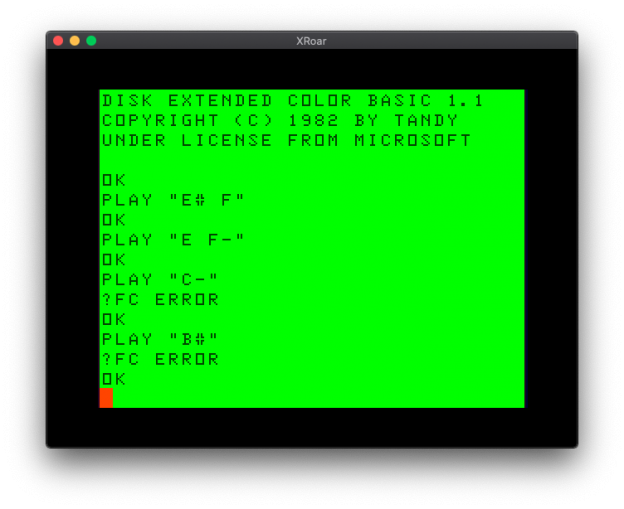# Extended Color BASIC plays non-existent notes.

Just for fun…

When I was working on my SirSound project, I had to create my own implementation of the Extended Color BASIC “PLAY” command. I did this by going through the Extended BASIC Unravelled book and looking at the 6809 assembly for the command.

I made a workalike function in C for the Arduino:

https://github.com/allenhuffman/PlayParser

…and while doing so, discovered an interesting thing about the PLAY parser and how it handled the notes.

The PLAY command operates by reading a command followed by an option modifying. For instance, “V10” is the command “V” for volume, and a modifier “10” for volume level 10. “T8” is command “T” for tempo and “8” for the speed.

When it comes to playing musical notes, PLAY understands the standard scale notes of C, D, E, F, G, A and B. When it parses a command letter, it then looks to see if a modifier is after it. The modifiers include “#” and “+” for sharp, and “-” for flat.

This allows you to play the full 12 note scale:

Using sharps: C C# D D# E F F# G G# A A# B (or C C+ D D+ E F F+ G G+ A A+ B)

Using flats: C D- D E- E F G- G A- A B- B

These notes correspond to the notes found on a piano keyboard:

I don’t understand music theory, but I know enough to say the white keys are the natural notes (CDEFGAB) and the black keys are a half step in between and are either sharps of the note before it (C# D# F# G# A#) or flats of the notes after it (Db Eb Gb Ab Bb). There is no E# or Fb or B# or Cb on a keyboard.

But, the BASIC PLAY parser does not add any code to prevent you from playing them anyway :-)

• PLAY “E# F” (E sharp and F natural) will play the same note.
• PLAY “E F-” (E natural and F flat) will play the same note.
• But you can’t PLAY “C-” (C flat) or “B#” (B sharp) because it knows to reject those modifiers from the notes at each end of the scale.

To me, this seems like a bug, but I implemented in my PLAY PARSER code anyway, just in case anyone used it. But since I lack any music theory background, I am probably wrong, as I have been told there is a reason for this.

For for someone like me with limited music background, it seemed like an odd thing to stumble upon in the code. I even documented in my SirSound documentation:

NOTE
—-
N (optional) followed by a letter from “A” to “G” or a number from 1 to 12.
When using letters, they can be optionally followed by “#” or “+” to make it
as sharp, or “-” to make it flat. When using numbers, they must be separated
by a “;” (semicolon).

C C# D D# E F F# G G# A A# B (sharps)
1 2 3 4 5 6 7 8 9 10 11 12
C D- D E- E F G- G A- A B- B (flats)

Due to how the original PLAY command was coded by Microsoft, it also allows
sharps and flats that would normally not be allowed. For instance, E# is the
same as F, and F- is the same a E. Since notes are numbered 1-12, the code
did not allow C- or B#. This quirk is replicated in this implementation.

SirSound documentation.

Ah, the things that amuse those of us without the brainpower to understand them.

Until next time…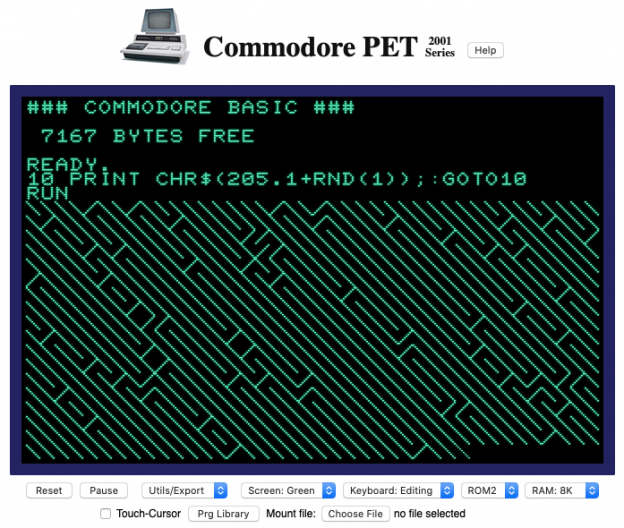# Porting 10 PRINT RACER by 8-Bit Show And Tell to the CoCo

YouTube decided to show me a video by 8-Bit Show And Tell. It is a BASIC driving game called “10 PRINT RACER” for the Commodore PET. For those that don’t know, “10 PRINT” is a one line Commodore BASIC program that generates a maze by randomly printing slash and backslash PETASCII characters.

`10 PRINT CHR\$(205.5+RND(1)); : GOTO 10`

There is even a book about it (which you can also download free). See the official site:

https://10print.org

I actually ported this to the CoCo years ago:

`10 PRINT CHR\$(47+(RND(2)-1)*45); : GOTO 10`

…but using the ASCII “/” and “\” characters just doesn’t have the same effect:

But I digress…

## 10 PRINT RACER

The game, 10 PRINT RACER, was based on this maze program. You drive a car through a passage in the middle of a random maze. The video demonstrates the game and then walks through the code, line by line.

I was impressed with his awareness of speeding up BASIC, such as using a FOR/NEXT loop with STEP 0 to create an infinite loop that is faster than using a GOTO. He also removes unnecessary spaces and combines lines together for faster execution. Heck, he even knows about using a period as a faster way to make zero!

## My PET experience

I’ve actually written programs for the PET. My high school had some, and I wrote a banner printing program for my typing teacher. She’d already written PRINT statements for each large letter based on needlepoint patterns, but there wasn’t enough memory in the PET to load them at the same time.

I solved this problem by having a main program input the message to print, then POKEing it to screen memory along with a “which character are we printing” counter. If the character to print was not in the current program, it would load and run the one that contained it. Those programs would then PEEK that screen memory to see what character it was supposed to print next.

A klunky solution, but it worked. (And in case you wondered why I used screen memory… I did not have a PET memory map, so I didn’t know where I could store the message data. I knew I could find screen memory by printing something on the screen and then PEEKing through memory until I found it. Once I located where the screen was stored, I used that for temporary memory. Funny enough, I did the same thing years later for my *ALLRAM* BBS when I needed a safe spot to temporarily load and execute PCLEAR 0 code.)

But I digress… Again.

## From Commodore PET to Radio Shack CoCo

Inspired by the video, I decided to port the game over to Color BASIC. This involved a few things:

1. Screen Size: The PET screen is 40×25, so I had to scale everything down to fit on the CoCo’s 32×16 screen.
2. PETASCII: The game uses two special characters which are not part of the standard ASCII that the CoCo has. I substituted with a forward slash (“/”) and a back slash (“\”). It doesn’t look as maze-like as the PET version, but it is the same general idea. There is also a PETASCII character that you print to clear the screen. I replaced that with CLS.
3. Input: Commodore uses GET instead of INKEY\$. I changed “GET A\$” to “A\$=INKEY\$”.
4. Memory Map: The game uses POKE and PEEK to put the racer on the screen, and check to see if it hit something. I had to change the PET memory location to be 1024, the start of the CoCo screen. I was surprised to hear him say the Commodore 64’s screen also starts at 1024.
5. Spaces: Commodore BASIC has the SPC() keyword which prints that many spaces. Color BASIC has TAB() but it moves to columns, so it couldn’t be used. I created an S\$ of 32 spaces using STRING\$() and then used MID\$() to get just the number of them I needed. This is likely much slower than if I had SPC().
6. Controls: The PET has a numeric keyboard, so the PET version used 4 for left, and 6 for right. The CoCo has arrow keys, so I changed it to use the left and right arrow keys. It now looks for CHR\$(8) for left and CHR\$(9) for right. Parsing CHR\$ is slower than the original which looked for “4” and “6”.
7. Random Differences: I thought I was going to have to change the random numbers. The Commodore version of RND would return a value from 0 to 1. You would then multiply the result by a number (X) which gave you a number from 0 to X. On the CoCo, doing RND(X) would return 1 to X. But, I realized RND(0) on the CoCo would do the same thing, so I ended up not changing it.
8. Key Repeat: The Commodore has key repeat and a small typeahead buffer. At the end of the PET code there was a POKE to clear out that buffer so it wouldn’t instantly restart the game if key presses were still in the buffer. I removed that POKE since the CoCo has no such buffer.

Here is what I came up with:

And here is the code:

```0 ' 10 PRINT RACER
1 ' BY WWW.8BITSHOWANDTELL.COM
2 '
3 ' PORTED FROM PET TO COCO
4 ' BY SUBETHASOFTWARE.COM
5 R\$="":CLS:PRINT"INIT:";:FORX=1TO75:M\$=CHR\$(47+45*(RND(2)-1)):R\$=R\$+M\$:PRINTM\$;:NEXT
6 S\$=STRING\$(32," ")
10 CLS:C=16:R=10:W=12:D=0:S=1024
20 L=0:FORZ=0TO1STEP0:X=RND(.)*10
30 IFX<4THENR=R-1:IFR<1THENR=1
40 IFX>5THENR=R+1:IFR+W>29THENR=29-W
50 RN=RND(.)*28+1:PRINTMID\$(R\$,RN,R);MID\$(S\$,1,W);MID\$(R\$,RN,31-R-W)
60 D=D+1:L=L+1:IFL>49THENL=0:W=W-1:IFW<3THENW=3
70 IFD<16THENNEXT
75 A\$=INKEY\$:IFA\$=CHR\$(8)THENC=C-1
80 IFA\$=CHR\$(9)THENC=C+1
90 P=PEEK(S+C):IFP<>96THEN200
100 POKES+C,106:NEXT
200 PRINTTAB(13)"CRASH!":IFD>H THENH=D
205 PRINTTAB(6)"SCORE:"D"  HIGH:"H
210 FORX=1TO2000:NEXT:A\$=INKEY\$
220 A\$=INKEY\$:IFA\$=""THEN220
230 GOTO10```

I tried to keep the code as close to the original as I could, so there are some more optimizations that we could do on the CoCo. For instance:

• Instead of using “RND(0)*10” for a value of 0-9, it might be faster to do RND(10) and adjust the IF/THEN to look for 1-10 instead of 0-9. This would save the overhead of doing the multiplication.
• If Extended BASIC can be used, then most constants can be changed to HEX values and they will be slightly faster. (Thought after seeing some code from Jim Gerrie recently, it may still be faster to parse some single digits as decimals over their HEX version. More on this in a future article.)
• Line 30 and 40 could be combined using ELSE, saving time each time the value is less than 5. Currently, if X is 1, it processes line 40 and then goes to line 50 which checks X again. Using ELSE would at least omit that step in that condition.
• The screen position variable (S) gets the car position (C) added to it each time it is POKEd or PEEKed. To save that math, the position of the car could be initialized as S+C and the two “+C”s in the code could be removed.
• There is a special IF in line 70 that is used to initially draw the screen before letting the car drive on it. Once the screen is drawn, it still checks this IF every time through. Time could be saved by drawing the initial screen outside of the main loop and then not needing to check that again.

What else do you see that might help speed things up? Please leave your comments, or take this code and see what you can do with it.

## PET emulator online

If you never got the pleasure of using one of these early Commodore Business Machines, I found a PET emulator that runs from a web browser:

https://www.masswerk.at/pet/

You can use that to type in the original Commodore “10 PRINT” program and see it run it all its glory:

Until next time…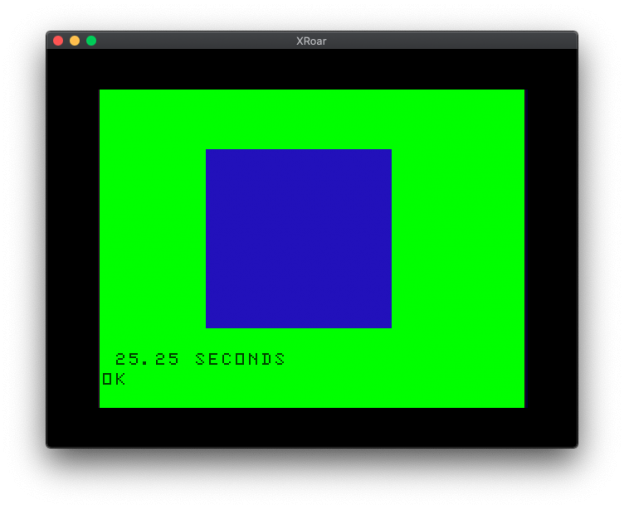# Color BASIC optimization challenge – more attempts

The prolific Jim Gerrie has ported the original scaling demo over to the MC-10 and optimized it. On his system, it reports 9.5 seconds!

He shared his code to his github page, but I’ll include it here for commentary:

```0 REM scale.bas
1 GOSUB100:TM=TIMER:FORZ=1TO M:CLS:A\$=STRING\$(W,C):FORA=(8-INT(HB))L+E-INT(WB)TOHL STEPL:PRINT@A,A\$:NEXT:IFH<1ORH>=&H10 THENQ=-Q:R=-R
2 W=W+Q:H=H+R:NEXT
3 REM 60=NTSC 50=PAL
4 T=TIMER:PRINT:PRINT (T-TM)/60;"SECONDS"
5 END
100 DIMW,H,A\$,A,B,L,Q,R,C,E,M,Z
110 I=32/4:REM SCALE WIDTH
120 J=16/3:REM SCALE HEIGHT
130 D=.1:REM SCALE INC/DEC
140 S=.5:REM SCALE FACTOR
150 W=IS:H=JS
160 Q=ID:R=JD
170 L=32:M=&H64
180 B=1/2:C=&HAF:E=15
190 RETURN```

Not prepared to let an MC-10 beat a CoCo, I wanted to try it myself on my test system, the Xroar emulator.

I did. And I got 10.75 seconds! The CoCo is slower than the MC-10?

But that’s still faster than our previous best attempt of 13.3 seconds by Xroar author Ciaran Anscomb. Maybe I can speed the CoCo up a bit. Someone commented that DISK BASIC was slightly slower due to hooking in to an interrupt (I think it uses that for a time delay when turning off the drive motor after disk access). Since the MC-10 doesn’t have DISK anyway, I thought I’d disable RS-DOS and try it again.

11.78 seconds without Disk BASIC. IT got even slower? That’s odd. I tried this last night on the Mac Xroar emulator and thought it was slightly faster.

We have seen variances between emulators and systems when it comes to timing, so at some point we need to find a better way to do this. I mean, the MC-10 can’t be faster, can it?

Speaking of the MC-10, first, you should be aware that JIm Gerrie is one of the most prolific programmers around, porting and writing software on what seems to be a daily basis. Just check out his YouTube channel sometime. He has an incredible version of the Rally-X arcade game, entirely in BASIC.

But I digress.

I want to point out that Jim normally wouldn’t have been able to run my scaling demo on an MC-10 since it does not include a TIMER function, nor does it support HEX numbers (as far as I know?). He is using MCX BASIC by Darren Atkinson. Darren is the designer behind the CoCoSDC floppy disk replacement project. MCX-BASIC adds things like TIMER and HEX to the MC-10, making it closer to Extended Color BASIC on the CoCo.

But I digress again.

In the previous article, Adam shared the results of his version, and has now posted his code:

```1 DIM SW,SH,SM,S,TM,Z,W,H,P
2 DIM A,B,C,D,E,F,L\$
3 SW=8:SH=5.33333334:SM=.1:S=.5
4 B=32:C=175:D=15:E=2:F=7
5 TM=TIMER
6 FORZ=1TO100
7 W=INT(SW*S):H=INT(SH*S)
8 P=D-INT(W/E)+(F-INT(H/E))*B
9 L\$=STRING\$(W,C)
10 CLS
11 FORA=1TO H:PRINT@P+A*B,L\$:NEXT
12 S=S+SM
13 IF H<1 OR H>D THEN SM=-SM:S=S+(SM*E)
14 NEXT
15 PRINT:PRINT (TIMER-TM)/60;”SECONDS”```

On my Xroar CoCo 2 test platform I get 17.93 seconds. Adam also sent in an interesting note which may explain some of the timing differences I am seeing reported:

This exercise also highlights the speed differences between a Coco2 and Coco3. I think the GIME chip is slower than the VDG in printing to the low-res screen. A Coco3 runs this code roughly 2 seconds slower!

Now that is an interesting observation. When I got my CoCo 3, my old machine went back in the box and I never had them both hooked up at the same time to do any comparisions.

I knew that the CoCo 3 40/80 column screens seemed slower. There are patches floating around that speed them up dramatically. Apparently it does some kind of MMU memory bank switch in and out for each character displayed. I did not realize there would be any difference in the 32 column VDG style screen. I’ll have to look into this and see if I can find out why.

## Walter Zambotti

On the CoCo mailing list (if you use e-mail, and like the CoCo, you should sign up), Australian Walter Zambotti saw the original example and provided a tip:

Try changing the inner loop to remove all calculations like this

115 P2-P+32:H2=P*H+32:BK\$=STRING\$(W,175)
120 FOR A=P2 TO H2 STEP 32
130 PRINT @A,BK\$
140 NEXT A

I believe I chopped 7 seconds off the time.

Walter Zambotti via CoColist on March 13, 2020

It seems others picked up on this as well, as I have seen some speedy attempts that pre-calculate values (so the FOR/NEXT loop only has to increment by 32 to get to the next line for PRINT) and pre-render the string of blue blocks. (I was aware of strings being quite slow after my String Theory experiments, but some of the pre-calculated values I would not have thought of.)

Nice job, Walter!

## Mission: Beat the MC-10

This leaves us with a problem. Jim Gerrie’s MC-10 version is still the fastest. Perhaps the 6800 in the MC-10 and it’s BASIC is just faster. Perhaps Jim’s just better at BASIC than we are. I’m willing to accept the second part, but my pride doesn’t want the first part to be true.

Can you make this faster than what Jim did? With the various attempts shared so far, perhaps bits and pieces of each of them could be combined to create something even faster?

Here is the original un-optimized code again for reference:

```0 REM scale.bas
10 SW=32/4 ' SCALE WIDTH
20 SH=16/3 ' SCALE HEIGHT
30 SM=.1 ' SCALE INC/DEC
40 S=.5 ' SCALE FACTOR
70 TM=TIMER:FOR Z=1 TO 100
80 W=INT(SWS) 90 H=INT(SHS)
100 P=15-INT(W/2)+(7-INT(H/2))32 110 CLS 120 FOR A=1 TO H 130 PRINT@P+A32,STRING\$(W,175)
140 NEXT A
150 S=S+SM
160 IF H<1 OR H>15 THEN SM=-SM:S=S+(SM*2)
170 NEXT Z
180 ' 60=NTSC 50=PAL
190 PRINT:PRINT (TIMER-TM)/60;"SECONDS"```

If you don’t have access to a real CoCo or emulator, you could use one of these from a web browser:

Although there is a way to load code into them, I am not sure if there is a way to get the code back out. However, I have been typing my BASIC up in a text editor on my Mac. Xroar allows mounting a test file (with the extension of .bas or .asc) as a cassette tape, then doing a “CLOAD” to load it in as if it were a program saved to tape in ASCII format. This allows me to edit and make changes on my Mac, then load the results into Xroar for testing.

If you try Xroar Online, set the “Machine:” type to “Tandy CoCo (NTSC)” to match the timing of the emulated Amercian CoCo I am using (where TIMER is 60 tickts per second, versus the PAL version that is 50 per second). Then, save out the code as a text file and mount it using the “Tape:” insert option. You can then type CLOAD in the emulator to load and RUN it.

Any takers?

Until next time…

# Obfuscated CoCo Color BASIC code is fun.

```10 A=26493:B=66:C=13:GOSUB40
20 A=291227:B=B+2:C=C+3:GOSUB40
30 END
40 I=RND(-A):FOR I=1 TO 4:PRINT CHR\$(B+RND(C));:NEXT:RETURN```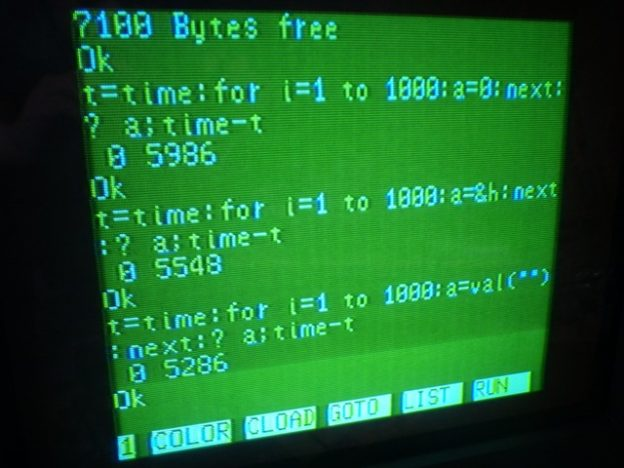# The fastest way to zero in Color BASIC.

In my Optimizing Color BASIC series, one of the things I learned was how much faster it was to use HEX values instead of decimal values. For example:

```A=65535
A=&HFFFF```

Even though the second statement is one character more to parse, it is still much faster since it’s easier for the interpreter to calculate base-16 values than base-10. This was even the case for zero:

```A=0
A=&H0```

Somewhere in the comments, CoCoSDC creator Darren Atkinson let me know about using just a period for zero. I wrote tested that in part 9.

Recently (March 28), Carlos Comacho tagged me in the Facebook CoCo group about something he found on a NEC PC-6001 Z-80 computer testing various ways to set something to zero. Here is the screenshot he shared:

Using a decimal 0 and HEX &H0 were tested, as well as using VAL(“”). Huh?

In the CoCo 3 BASIC quick reference guide, I find this entry:

I am aware of using this to convert a STRING to a number, such as when using LINE INPUT:

```10 LINE INPUT "ENTER YOUR AGE:";A\$
20 A=VAL(A\$)
30 IF A=42 THEN PRINT "ULTIMATE AGE!"```

It also handles decimal values, such as A=VAL(“12.34”). And I guess I knew that if you passed it an empty string, it would return zero because pressing ENTER on a LINE INPUT prompt that then went into VAL would return 0…

## Let’s do nothing!

Of course I had to benchmark this and see what CoCo did with it! Here’s the current version of my BASIC benchmark test:

```0 REM BENCH0.BAS
5 DIM TE,TM,B,A,TT
10 FORA=0TO3:TIMER=0:TM=TIMER
20 FORB=0TO1000
30 Z=0
70 NEXT
80 TE=TIMER-TM:PRINTA,TE
90 TT=TT+TE:NEXT:PRINTTT/A:END```

Running this produces a value of 189. Next we try HEX &H0:

```0 REM BENCHX0.BAS
5 DIM TE,TM,B,A,TT
10 FORA=0TO3:TIMER=0:TM=TIMER
20 FORB=0TO1000
30 Z=&H0
70 NEXT
80 TE=TIMER-TM:PRINTA,TE
90 TT=TT+TE:NEXT:PRINTTT/A:END```

That shows 174. Now let’s do the one with the period:

```0 REM BENCHDOT.BAS
5 DIM TE,TM,B,A,TT
10 FORA=0TO3:TIMER=0:TM=TIMER
20 FORB=0TO1000
30 Z=.
70 NEXT
80 TE=TIMER-TM:PRINTA,TE
90 TT=TT+TE:NEXT:PRINTTT/A:END```

That one gives me 147 – the fastest so far! And lastly, the silly VAL(“”) empty quote thing:

```0 REM BENCHVAL.BAS
5 DIM TE,TM,B,A,TT
10 FORA=0TO3:TIMER=0:TM=TIMER
20 FORB=0TO1000
30 Z=VAL("")
70 NEXT
80 TE=TIMER-TM:PRINTA,TE
90 TT=TT+TE:NEXT:PRINTTT/A:END```

This one gives me 212, even slower than decimal 0.

It looks like, on Color BASIC at least, the VAL(“”) trick is not useful, but I appreciate Carlos letting me know about it.

Have you seen this on any other flavor of BASIC? Let me know in the comments.

Until next time…

# Random BASIC shuffling revisited.

In a previous article, I wrote a BASIC program that simulated pulling marbles out of a bag. I described my thought process, including how I initially thought I would have to randomize the virtual marbles in the virtual bag. I realized this would be unnecessary, but still offered a demonstration of a simple way to randomly shuffle an array of items, as one might do with a deck of playing cards:

NOTE: I had a bug on line 40 and was only counting from 0 to 24 (25 letters) instead of 0 to 25 (26 letters of the alphabet). Fixed here:

```10 REM shuffle.bas
20 REM CREATE ALPHABET ARRAY
30 DIM A\$(25)
40 FOR A=0 TO 25
50 A\$(A)=CHR\$(65+A)
60 NEXT

70 REM DISPLAY ARRAY
80 GOSUB 190

90 REM SWAP EACH ONE RANDOMLY
100 FOR A=0 TO 25
110 S=RND(26)-1
120 SV\$=A\$(S)
130 A\$(S)=A\$(A)
140 A\$(A)=SV\$
150 NEXT

160 REM DISPLAY ARRAY
170 GOSUB 200

180 END

190 REM DISPLAY ARRAY
200 FOR A=0 TO 25
210 PRINT A\$(A);
220 NEXT
230 PRINT
240 RETURN```

That would display the 26 letters of the alphabet, then shuffle them and display the results. My shuffle routine went through each position (0 to 25) and swapped it with another random position (0 to 25).

However, adding to the flaw in the simulation that article was about, there was another flaw in my shuffling examples.

In a comment, James Jones added:

Actually, to get a random shuffle with all permutations equally likely, you have to do something like this:

FOR i:= 1 to n-1
j:=i+INT(RND(n+1-i))
temp:=a(i)\a(i):=a(j)\a(j):=temp
NEXT i

i.e. at each step you swap a(i) with a(j) where j is a randomly chosen number between i and n inclusive.

James Jones

His program logic uses ‘n’ as the number of elements we are shuffling, which is 26 for my example.

The for/next loop of ‘i’ will count from 1 to n-1, so 1 to 25 in this example.

‘j’ will be the randomly selected target element to swap with the element at ‘i’. It is chosen using the random number generator. I it looks like his rnd(26) would return 0-25, so the result of j for each count of 1 to n-1 would be

```i = 1 -> j = 1 + rnd(26 + 1 - 1) -> j = 1 + rnd(26) -> j = 1 to 26
i = 2 -> j = 2 + rnd(26 + 1 - 2) -> j = 2 + rnd(25) -> j = 2 to 26
i = 3 -> j = 3 + rnd(26 + 1 - 3) -> j = 3 + rnd(24) -> j = 3 to 26
...
i = 23 -> j = 23 + rnd(26 + 1 - 23) -> j = 23 + rnd(4) -> j = 23 to 26
i = 24 -> j = 24 + rnd(26 + 1 - 24) -> j = 24 + rnd(3) -> j = 24 to 26
i = 25 -> j = 25 + rnd(26 + 1 - 25) -> j = 25 + rnd(2) -> j = 25 to 26```

It then uses a temporary variable to swap the position of a(i) with a(j).

My original version randomly swapped each position with any of the 26 positions. James’ code swaps each position with only positions after it.

With apologies to James for down-porting his logic to Microsoft BASIC, here is my original example updated to take this approach. To keep it as close to my original example as possible, I’ll adjust my array to use 1-26 (instead of 0-25). Color BASIC arrays are base-0, but for this example we’ll just ignore the zero. I’ll try to bold the changes from the original.

```10 REM jjshuffle.bas
20 REM CREATE ALPHABET ARRAY
25 N=26
30 DIM A\$(N)
40 FOR A=1 TO N
50 A\$(A)=CHR\$(64+A)
60 NEXT

70 REM DISPLAY ARRAY
80 GOSUB 190

90 REM SWAP EACH ONE RANDOMLY
100 FOR I=1 TO N-1
110 J=I+INT(RND(N+1-I)-1)
120 TEMP\$=A\$(I)
130 A\$(I)=A\$(J)
140 A\$(J)=TEMP\$
150 NEXT

160 REM DISPLAY ARRAY
170 GOSUB 200

180 END

190 REM DISPLAY ARRAY
200 FOR A=1 TO N
210 PRINT A\$(A);
220 NEXT
230 PRINT
240 RETURN```

And to convert this back to my first example using base-0 arrays:

```10 REM shuffle2.bas
20 REM CREATE ALPHABET ARRAY
30 DIM A\$(25)
40 FOR A=0 TO 24
50 A\$(A)=CHR\$(65+A)
60 NEXT

70 REM DISPLAY ARRAY
80 GOSUB 190

90 REM SWAP EACH ONE RANDOMLY
100 FOR A=0 TO 25
110 S=A+RND(26-A)-1
115 PRINT A,S
120 SV\$=A\$(S)
130 A\$(S)=A\$(A)
140 A\$(A)=SV\$
150 NEXT

160 REM DISPLAY ARRAY
170 GOSUB 200

180 END

190 REM DISPLAY ARRAY
200 FOR A=0 TO 25
210 PRINT A\$(A);
220 NEXT
230 PRINT
240 RETURN```

Any other random thoughts?

Until next time…